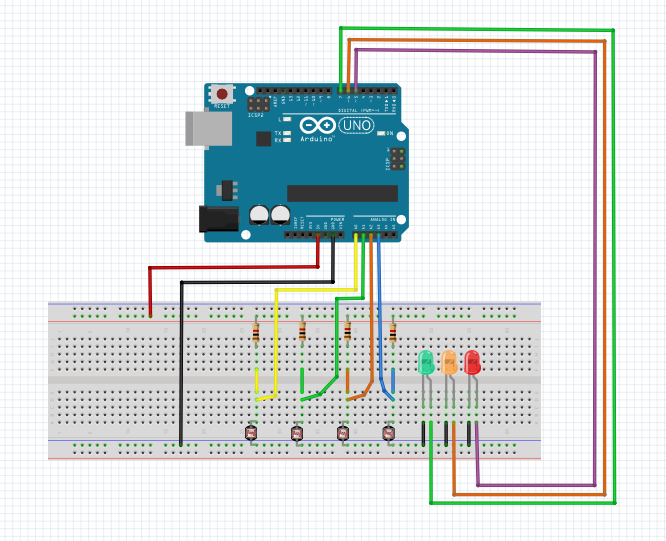# B4R TutorialCheck car's lights status and report

Hey everyone,

here is an implementation of a car lights checker which reports if all 4 car's lights are working properly. To find out if there is a problem with the lights (head and rear) we use Light Dependent Resistors (LDR). We form 4 voltage dividers with the LDR positioned in such a way that more light means less voltage and less light means more voltage in the analog read pins. Reading the values of the analog read pins A0-A3 we process the results and light up the corresponding led.

Here is the schematic and the code:B4R Code:
``````#Region Project Attributes
#AutoFlushLogs: True
#CheckArrayBounds: True
#StackBufferSize: 300
#End Region
'Ctrl+Click to open the C code folder: ide://run?File=%WINDIR%\System32\explorer.exe&Args=%PROJECT%\Objects\Src

Sub Process_Globals
Public Serial1 As Serial

Dim ledR As Pin
Dim ledO As Pin
Dim ledG As Pin

Dim vFL As Pin
Dim vFR As Pin
Dim vBL As Pin
Dim vBR As Pin

Dim previousValue As UInt
End Sub

Private Sub AppStart
Serial1.Initialize(115200)
Log("AppStart")

ledR.Initialize(5, ledR.MODE_OUTPUT)
ledO.Initialize(6, ledO.MODE_OUTPUT)
ledG.Initialize(7, ledG.MODE_OUTPUT)

vFL.Initialize(vFL.A3, vFL.MODE_INPUT)
vFR.Initialize(vFR.A2, vFR.MODE_INPUT)
vBL.Initialize(vBL.A1, vBL.MODE_INPUT)
vBR.Initialize(vBR.A0, vBR.MODE_INPUT)

End Sub

Sub CheckLDRResistance

'Change the levels accordingly
Dim levelF_2 As UInt = 800
Dim levelF_1 As UInt = 400
Dim levelB_2 As UInt = 700
Dim levelB_1 As UInt = 350

Dim valueFL As UInt = vFL.AnalogRead
Dim valueFR As UInt = vFR.AnalogRead
Dim valueBL As UInt = vBL.AnalogRead
Dim valueBR As UInt = vBR.AnalogRead

'ValueFL
Select Case True
Case (valueFL < levelF_1)
valueFL = 10
Case (valueFL > levelF_1 And valueFL < levelF_2)
valueFL = 5
Case (valueFL > levelF_2)
valueFL = 0
End Select

'ValueFR
Select Case True
Case (valueFR < levelF_1)
valueFR = 10
Case (valueFR > levelF_1 And valueFR < levelF_2)
valueFR = 5
Case (valueFR > levelF_2)
valueFR = 0
End Select

'ValueBL
Select Case True
Case (valueBL < levelB_1)
valueBL = 10
Case (valueBL > levelB_1 And valueBL < levelB_2)
valueBL = 5
Case (valueBL > levelB_2)
valueBL = 0
End Select

'ValueBR
Select Case True
Case (valueBR < levelB_1)
valueBR = 10
Case (valueBR > levelB_1 And valueBR < levelB_2)
valueBR = 5
Case (valueBR > levelB_2)
valueBR = 0
End Select

Dim currentValue As UInt = valueBR + valueBL + valueFL + valueFR

Select Case True
Case currentValue <= 10  '2 lights are off at least
If previousValue <> currentValue Then
ResetLEDValues
ledR.DigitalWrite(True)
previousValue = currentValue
End If
Case (currentValue > 10  And currentValue < 20) '1 lights is off
If previousValue <> currentValue Then
ResetLEDValues
ledO.DigitalWrite(True)
previousValue = currentValue
End If
Case (currentValue >= 20  And currentValue <= 40) 'Everything seems to work properly
'- check if front and back values are the same on each side
If previousValue <> currentValue Then
ResetLEDValues
If valueFL <> valueFR Or valueBL <> valueBR Then
ledO.DigitalWrite(True)
Else
ledG.DigitalWrite(True)
End If
previousValue = currentValue
End If
End Select
End Sub

Sub ResetLEDValues
ledR.DigitalWrite(False)
ledO.DigitalWrite(False)
ledG.DigitalWrite(False)
End Sub``````

Last edited:
•JohnC, José J. Aguilar and Erel